NameFlagsCard.TypeDescription & Constraints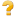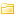Extension 0..1ExtensionReason for current status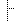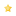extension 0..0url 1..1uri"http://hl7.org/fhir/StructureDefinition/request-statusReason"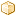value[x] 1..1CodeableConceptValue of extension
Binding: (unbound) (example): Codes identifying the reason for the current state of an event.Documentation for this format
<status value="active"/> <experimental value="false"/> <date value="2017-12-06"/> <publisher value="HL7 International / FHIR Infrastructure"/> <contact> <telecom> <system value="url"/> <value value="http://www.hl7.org/Special/committees/fiwg"/> </telecom> </contact> <description value="Captures the reason for the current state of the resource."/> <jurisdiction> <coding> <system value="http://unstats.un.org/unsd/methods/m49/m49.htm"/> <code value="001"/> </coding> </jurisdiction> <fhirVersion value="5.0.0"/> <mapping> <identity value="v2"/> <uri value="http://hl7.org/v2"/> <name value="HL7 v2 Mapping"/> </mapping> <mapping> <identity value="rim"/> <uri value="http://hl7.org/v3"/> <name value="RIM Mapping"/> </mapping> <kind value="complex-type"/> <abstract value="false"/> <context> <type value="element"/> <expression value="DeviceRequest"/> </context> <context> <type value="element"/> <expression value="ServiceRequest"/> </context> <context> <type value="element"/> <expression value="NutritionOrder"/> </context> <context> <type value="element"/> <expression value="SupplyRequest"/> </context> <type value="Extension"/> <baseDefinition value="http://hl7.org/fhir/StructureDefinition/Extension"/> <derivation value="constraint"/> <snapshot> <element id="Extension"> <path value="Extension"/> <short value="Reason for current status"/> <definition value="Captures the reason for the current state of the resource."/> <comment value="This is generally only used for "exception" statuses such as "suspended" or "cancelled". The reason for performing the request at all is captured in reasonCode, not here. (Distinct reason codes for different statuses can be enforced using invariants if they are universal bindings)."/> <min value="0"/> <max value="1"/> <base> <path value="Extension"/> <min value="0"/> <max value="*"/> </base> <constraint> <key value="ele-1"/> <severity value="error"/> <human value="All FHIR elements must have a @value or children"/> <expression value="hasValue() or (children().count() > id.count())"/> <source value="http://hl7.org/fhir/StructureDefinition/Element"/> </constraint> <constraint> <key value="ext-1"/> <severity value="error"/> <human value="Must have either extensions or value[x], not both"/> <expression value="extension.exists() != value.exists()"/> <source value="http://hl7.org/fhir/StructureDefinition/Extension"/> </constraint> <isModifier value="false"/> <mapping> <identity value="v2"/> <map value="Varies by domain"/> </mapping> <mapping> <identity value="rim"/> <map value=".inboundRelationship[typeCode=SUBJ].source[classCode=CACT, moodCode=EVN].reasonCOde"/> </mapping> </element> <element id="Extension.id"> <path value="Extension.id"/> <representation value="xmlAttr"/> <short value="Unique id for inter-element referencing"/> <definition value="Unique id for the element within a resource (for internal references). This may be any string value that does not contain spaces."/> <min value="0"/> <max value="1"/> <base> <path value="Element.id"/> <min value="0"/> <max value="1"/> </base> <type> <extension url="http://hl7.org/fhir/StructureDefinition/structuredefinition-fhir-type"> <valueUrl value="id"/> </extension> <code value="http://hl7.org/fhirpath/System.String"/> </type> <condition value="ele-1"/> <isModifier value="false"/> <isSummary value="false"/> <mapping> <identity value="rim"/> <map value="n/a"/> </mapping> </element> <element id="Extension.extension"> <path value="Extension.extension"/> <slicing> <discriminator> <type value="value"/> <path value="url"/> </discriminator> <description value="Extensions are always sliced by (at least) url"/> <rules value="open"/> </slicing> <short value="Extension"/> <definition value="An Extension"/> <min value="0"/> <max value="0"/> <base> <path value="Element.extension"/> <min value="0"/> <max value="*"/> </base> <type> <code value="Extension"/> </type> <constraint> <key value="ele-1"/> <severity value="error"/> <human value="All FHIR elements must have a @value or children"/> <expression value="hasValue() or (children().count() > id.count())"/> <source value="http://hl7.org/fhir/StructureDefinition/Element"/> </constraint> <constraint> <key value="ext-1"/> <severity value="error"/> <human value="Must have either extensions or value[x], not both"/> <expression value="extension.exists() != value.exists()"/> <source value="http://hl7.org/fhir/StructureDefinition/Extension"/> </constraint> <isModifier value="false"/> <isSummary value="false"/> </element> <element id="Extension.url"> <path value="Extension.url"/> <representation value="xmlAttr"/> <short value="identifies the meaning of the extension"/> <definition value="Source of the definition for the extension code - a logical name or a URL."/> <comment value="The definition may point directly to a computable or human-readable definition of the extensibility codes, or it may be a logical URI as declared in some other specification. The definition SHALL be a URI for the Structure Definition defining the extension."/> <min value="1"/> <max value="1"/> <base> <path value="Extension.url"/> <min value="1"/> <max value="1"/> </base> <type> <extension url="http://hl7.org/fhir/StructureDefinition/structuredefinition-fhir-type"> <valueUrl value="uri"/> </extension> <code value="http://hl7.org/fhirpath/System.String"/> </type> <fixedUri value="http://hl7.org/fhir/StructureDefinition/request-statusReason"/> <isModifier value="false"/> <isSummary value="false"/> <mapping> <identity value="rim"/> <map value="N/A"/> </mapping> </element> <element id="Extension.value[x]"> <path value="Extension.value[x]"/> <short value="Value of extension"/> <definition value="Value of extension - must be one of a constrained set of the data types (see [Extensibility](http://hl7.org/fhir/R5/extensibility.html) for a list)."/> <min value="1"/> <max value="1"/> <base> <path value="Extension.value[x]"/> <min value="0"/> <max value="1"/> </base> <type> <code value="CodeableConcept"/> </type> <condition value="ext-1"/> <constraint> <key value="ele-1"/> <severity value="error"/> <human value="All FHIR elements must have a @value or children"/> <expression value="hasValue() or (children().count() > id.count())"/> <source value="http://hl7.org/fhir/StructureDefinition/Element"/> </constraint> <isModifier value="false"/> <isSummary value="false"/> <binding> <extension url="http://hl7.org/fhir/tools/StructureDefinition/binding-definition"> <valueMarkdown value="Codes identifying the reason for the current state of an event."/> </extension> <extension url="http://hl7.org/fhir/StructureDefinition/elementdefinition-bindingName"> <valueString value="StatusReason"/> </extension> <strength value="example"/> <description value="Codes identifying the reason for the current state of an event."/> </binding> <mapping> <identity value="rim"/> <map value="N/A"/> </mapping> </element> </snapshot> <differential> <element id="Extension"> <path value="Extension"/> <short value="Reason for current status"/> <definition value="Captures the reason for the current state of the resource."/> <comment value="This is generally only used for "exception" statuses such as "suspended" or "cancelled". The reason for performing the request at all is captured in reasonCode, not here. (Distinct reason codes for different statuses can be enforced using invariants if they are universal bindings)."/> <min value="0"/> <max value="1"/> <mapping> <identity value="v2"/> <map value="Varies by domain"/> </mapping> <mapping> <identity value="rim"/> <map value=".inboundRelationship[typeCode=SUBJ].source[classCode=CACT, moodCode=EVN].reasonCOde"/> </mapping> </element> <element id="Extension.extension"> <path value="Extension.extension"/> <max value="0"/> </element> <element id="Extension.url"> <path value="Extension.url"/> <fixedUri value="http://hl7.org/fhir/StructureDefinition/request-statusReason"/> </element> <element id="Extension.value[x]"> <path value="Extension.value[x]"/> <min value="1"/> <type> <code value="CodeableConcept"/> </type> <binding> <extension url="http://hl7.org/fhir/tools/StructureDefinition/binding-definition"> <valueMarkdown value="Codes identifying the reason for the current state of an event."/> </extension> <extension url="http://hl7.org/fhir/StructureDefinition/elementdefinition-bindingName"> <valueString value="StatusReason"/> </extension> <strength value="example"/> <description value="Codes identifying the reason for the current state of an event."/> </binding> </element> </differential> </StructureDefinition>